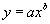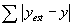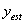POWER REGRESSION APPLET

This applet has two functions: plotting and finding the power function (of the form) which best approximates the user supplied data. Use it as follows:

1. Put the data to plot and analyze in the Data section. Though the variables default to x (the independent variable) and y (the dependent variable), they can be renamed. Renaming them will cause the graph to be updated. The values are entered one per line. You can also copy and paste data from any other application using the keys CTRL C to copy and CTRL V to paste.
2. The buttons work as follows: CLEAR will clear the data, the plot area and the RESULTS area. PLOT will plot the data as points. ANALYZE will find the power function which best approximates the data, plot it and display its equation in the RESULTS area.
3. The plot area (top, right) will show the plot.
4. The RESULTS area is used to communicate with the user. Errors will be displayed there, for example if the number of values is not the same for both variables. Information will also be displayed there. For example, when the user clicks on ANALYZE, the equation of the regression function will appear there, as well as the error associated with this function and the correlation coefficient. The error is obtained with the formulawhere y denotes the y-values of the data points to fit anddenotes the computed y-values.

This applet was developed by Dr. Philippe B. Laval, at Kennesaw State University.
This work was funded in part by:
The National Science Foundation grants # GEO-0355224 and # DUE-9952568
The US Department of Education FIPSE #P116B00178